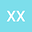•In this paper we study partial regularity of weak solutions to the initial boundary value problem for the system $-\textup{div}\left[(I+{\bf m}\otimes {\bf m})\nabla p\right]=S(x),\ \ \partial_t{\bf m}-D^2\Delta {\bf m}-E^2({\bf m}\cdot\nabla p)\nabla p+|{\bf m}|^{2(\gamma-1)}{\bf m}=0$, where $S(x)$ is a given function and $D, E, \gamma$ are given numbers. This problem has been proposed as a PDE model for biological transportation networks. The mathematical difficulty is due to the fact that the system in the model features both a quadratic nonlinearity and a cubic nonlinearity. The regularity issue seems to have a connection to a conjecture by De Giorgi \cite{DE}. We also investigate the life-span of classical solutions. Our results show that local existence of a classical solution can always be obtained and the life-span of such a solution can be extended as far away as one wishes as long as the term $\|{\bf m}(x,0)\|_{\infty, \Omega}+\|S(x)\|_{\frac{2N}{3}, \Omega}$ is made suitably small, where $N$ is the space dimension and $\|\cdot\|_{q,\Omega}$ denotes the norm in $L^q(\Omega)$.